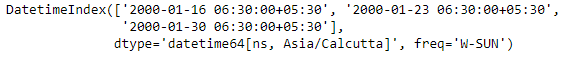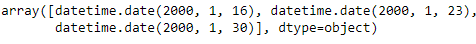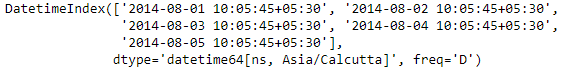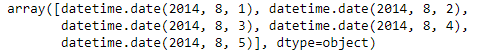# Python | Pandas DatetimeIndex.date

• Last Updated : 24 Dec, 2018

Python is a great language for doing data analysis, primarily because of the fantastic ecosystem of data-centric python packages. Pandas is one of those packages and makes importing and analyzing data much easier.

Pandas` DatetimeIndex.date` attribute outputs an Index object containing the date values present in each of the entries of the DatetimeIndex object.

Syntax: DatetimeIndex.date

Return: numpy array of python datetime.date

Example #1: Use `DatetimeIndex.date` attribute to find the date part of the DatetimeIndex object.

 `# importing pandas as pd``import` `pandas as pd`` ` `# Create the DatetimeIndex``# Here 'W' represents Weekly frequency``didx ``=` `pd.DatetimeIndex(start ``=``'2000-01-10 06:30'``, freq ``=``'W'``, ``                            ``periods ``=` `3``, tz ``=``'Asia/Calcutta'``)`` ` `# Print the DatetimeIndex``print``(didx)`

Output :Now we want to find all the date values present in the DatetimeIndex object.

 `# find all the date values present in the object``didx.date`

Output :As we can see in the output, the function has returned an Index object containing the date values present in each entry of the DatetimeIndex object.

Example #2: Use `DatetimeIndex.date` attribute to find the date part of the DatetimeIndex object.

 `# importing pandas as pd``import` `pandas as pd`` ` `# Create the DatetimeIndex``# Here 'D' represents Daily frequency``didx ``=` `pd.DatetimeIndex(start ``=``'2014-08-01 10:05:45'``, freq ``=``'D'``,``                               ``periods ``=` `5``, tz ``=``'Asia/Calcutta'``)`` ` `# Print the DatetimeIndex``print``(didx)`

Output :Now we want to find all the date values present in the DatetimeIndex object.

 `# find all the date values present in the object``didx.date`

Output :As we can see in the output, the function has returned an Index object containing the date values present in each entry of the DatetimeIndex object.

My Personal Notes arrow_drop_up